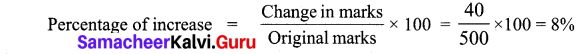# Samacheer Kalvi 7th Maths Solutions Term 3 Chapter 2 Percentage and Simple Interest Ex 2.3

Students can Download Maths Chapter 2 Percentage and Simple Interest Ex 2.3 Questions and Answers, Notes Pdf, Samacheer Kalvi 7th Maths Book Solutions Guide Pdf helps you to revise the complete Tamilnadu State Board New Syllabus and score more marks in your examinations.

## Tamilnadu Samacheer Kalvi 7th Maths Solutions Term 3 Chapter 2 Percentage and Simple Interest Ex 2.3

Question 1.
14 out of the 70 magazines at the bookstore are comedy magazines. What percentage of the magazines at the bookstore are comedy magazines?
Solution:
Total number of magazines in the bookstore = 100 m
Number of comedy magazines = 14
Percentage of comedy magzines = $$\frac { 14 }{ 70 }$$ × 100% = 20%
20% of the magazines are comedy magazines.

Question 2.
A tank can hold 50 litres of water. At present, it is only 30% full. How many litres of water will fill the tank, so that it is 50% full?
Solution:
Capacity of the tank = 50 litres
Amount of water filled = 30% of 50 litres = $$\frac { 30 }{ 100 }$$ × 50 = 15 litres
Amount of water to be filled = 50 – 15 = 35 litresQuestion 3.
Karun bought a pair of shoes at a sale of 25%. If the amount he paid was ₹ 1000, then find the marked price.
Solution:
Let the marked price of the raincoat be ₹ P
Amount he paid at a discount of 25% = ₹ 1000
(Marked Price) – (25% of P) = 1000
P – ($$\frac { 25 }{ 100 }$$ × P) = 1000
P – $$\frac { 1 }{ 4 }$$ × P = 1000
P (1 – $$\frac { 1 }{ 4 }$$) = 1000
$$\frac { 3 }{ 4 }$$ P = 1000
P = 1000 × $$\frac { 4 }{ 3 }$$
= $$\frac { 4000 }{ 3 }$$
P = 1333.33
∴ Marked price of the shoes = ₹ 1333

Question 4.
An agent of an insurance company gets a commission of 5% on the basic premium he collects. What will be the commission earned by him if he collects ₹ 4800?
Solution:
Commission earned = 5% of basic premium
Commission earned for ₹ 4800 = 5% of 4800
= $$\frac { 5 }{ 100 }$$ × 4800
= ₹ 240
Commission earned = ₹ 240

Question 5.
A biology class examined some flowers in a local Grass land. Out of the 40 flowers they saw, 30 were perennials. What percentage of the flowers were perennials?
Solution:
Number of flowers examined = 40
Number of perennials = 30
Percentage = $$\frac { 30 }{ 40 }$$ × 100%
= 75%
75% of the flowers were perennials.Question 6.
Solution:
Number of brown beads = 5
Percentage of brown beads = $$\frac { 15 }{ 50 }$$ × 100 %
= 10 %
10% of the beads was brown

Question 7.
Ramu scored 20 out of 25 marks in English, 30 out of 40 marks in Science and 68 out of 80 marks in mathematics. In which subject his percentage of marks is best?
Solution:
Ramu’s score in English = 20 out of 25
Percentage scored in English = $$\frac { 20 }{ 25 }$$ × 100 % = 80 %
Ramu’s Score in Science = 30 out of 40
Percentage scored in Science = $$\frac { 30 }{ 40 }$$ × 100 % = 75%
Ramu’s score in Mathematics = 68 out of 80
Percentage scored in Maths = $$\frac { 68 }{ 80 }$$ × 100 % = 85 %
85% > 80% > 75%.
∴ In Mathematics his percentage of marks is the best.Question 8.
Peter requires 50% to pass. If he gets 280 marks and falls short by 20 marks, what would have been the maximum marks of the exam?
Solution:
Peters score = 280 marks
Marks needed for a pass = 20
∴ Total marks required to get a pass = 280 + 20 = 300
i.e. 50% of total marks = 300
$$\frac { 50 }{ 100 }$$ × Total marks = 300
$$\frac { 1 }{ 2 }$$ × Total Marks = 300
Total Marks = 300 × 2 = 600
Total marks of the exam = 600

Question 9.
Kayal scored 225 marks out of 500 in revision test 1 and 265 out of 500 marks in revision test 2. Find the percentage of increase in her score.
Solution:
Marks scored in revision I = 225
Marks scored in revision II = 265
Change in marks = 265 – 225 = 40Percentage of increase in marks = 8%Question 10.
Roja earned ₹ 18,000 per month. She utilized her salary in the ratio 2 : 1 : 3 for education, savings and other expenses respectively. Express her usage of income in percentage.
Solution:
Amount of Salary = ₹ 18,000
(i) Total number of parts of salary = 2 + 1 + 3 = 6
Salary is divided into 3 portions as $$\frac { 2 }{ 6 }$$,$$\frac { 1 }{ 6 }$$ and $$\frac { 3 }{ 6 }$$
Portion of salary used for education = $$\frac { 2 }{ 6 }$$
Salary used for education = $$\frac { 2 }{ 6 }$$ × 18,000 = ₹ 6,000
Percentage for Education = $$\frac { 6000 }{ 18000 }$$ × 100 = 33.33%

(ii) Usage of salary for savings = $$\frac { 1 }{ 6 }$$ × 18,000 = ₹ 3,000
Percentage for savings = $$\frac { 3000 }{ 18000 }$$ × 100 = 16.67 %

(iii) Usage of salary for other expenses = $$\frac { 3 }{ 6 }$$ × 18,000 = ₹ 9,000
Percentage for other expenses = $$\frac { 9000 }{ 18000 }$$ × 100 = 50 %